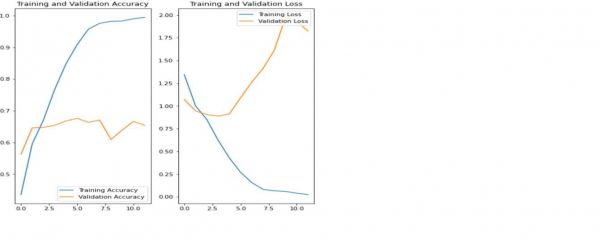# How can Tensorflow be used to visualize training results using Python?

The training results can be visualized with Tensorflow using Python with the help of the ‘matplotlib’ library. The ‘plot’ method is used to plot the data on the console.

We will use the Keras Sequential API, which is helpful in building a sequential model that is used to work with a plain stack of layers, where every layer has exactly one input tensor and one output tensor.

A neural network that contains at least one layer is known as a convolutional layer. We can use the Convolutional Neural Network to build learning model.

An image classifier is created using a keras.Sequential model, and data is loaded using preprocessing.image_dataset_from_directory. Data is efficiently loaded off disk. Overfitting is identified and techniques are applied to mitigate it. These techniques include data augmentation, and dropout. There are images of 3700 flowers. This dataset contains 5 sub directories, and there is one sub directory per class. They are: daisy, dandelion, roses, sunflowers, and tulips.

We are using the Google Colaboratory to run the below code. Google Colab or Colaboratory helps run Python code over the browser and requires zero configuration and free access to GPUs (Graphical Processing Units). Colaboratory has been built on top of Jupyter Notebook.

## Example

print("Calculating the accuracy")
acc = history.history['accuracy']
val_acc = history.history['val_accuracy']
print("Calculating the loss")
loss = history.history['loss']
val_loss = history.history['val_loss']

epochs_range = range(epochs)
print("The results are being visualized")
plt.figure(figsize=(8, 8))
plt.subplot(1, 2, 1)
plt.plot(epochs_range, acc, label='Training Accuracy')
plt.plot(epochs_range, val_acc, label='Validation Accuracy')
plt.legend(loc='lower right')
plt.title('Training and Validation Accuracy')
plt.subplot(1, 2, 2)

plt.plot(epochs_range, loss, label='Training Loss')
plt.plot(epochs_range, val_loss, label='Validation Loss')
plt.legend(loc='upper right')
plt.title('Training and Validation Loss')
plt.show()

## Output

Calculating the accuracy
Calculating the loss
The results are being visualized## Explanation

• The above plots indicate that the training accuracy and validation accuracy are not in sync.

• The model has achieved only about 60 percent accuracy on the validation dataset.

• This is known as overfitting.

• Training accuracy has increased linearly over time, but validation accuracy has stalled at around 60 percent in the training process.

• When the number of training examples is small, the model learns from noises or unwanted details from training examples.

• This negatively impacts the performance of the model on new examples.

• Due to overfitting, the model will not be able to generalize well on the new dataset.

• There are many ways in which overfitting can be avoided. We will use data augmentation to overcome overfitting.